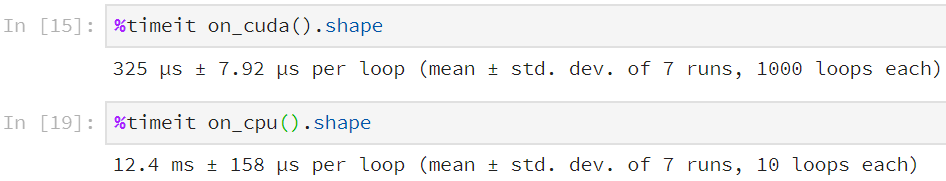## On-Disk Embeddings

##### August 4, 2018

There are some cases when you need to run your model on a small instance. For example, if your model is being called 1 time per hour or you just don’t want to pay \$150 per month to Amazon for t2.2xlarge instance with 32Gb RAM. The problem is that the size of most pre-trained word embeddings can reach tens of gigabytes.

In this post, I will describe the method of access word vectors without loading it into memory. The idea is to simply save word vectors as a matrix so that we could compute the position of each row without reading any other rows from disk.

Fortunately, all this logic is already implemented in numpy.memmap. The only thing we need to implement ourselves is the function which converts word into an appropriate index. We can simply store the whole vocabulary in memory or use hashing trick, it does not matter at this point.

It is slightly harder to store FastText vectors that way because it requires additional computation on n-grams to obtain word vector. So for simplicity, we will just pre-compute vectors for all necessary words.

You may take a look at a simple implementation of the described approach here: https://github.com/generall/OneShotNLP/blob/master/src/utils/disc_vectors.py

Class DiscVectors contains method for converting fastText .bin model into on-disk matrix representation and json file with vocabulary and meta-information. Once the model is converted, you can retrieve vectors with get_word_vector method. Performance check shows that in the worst case it takes 20 µs to retrieve single vector, pretty good since we are not using any significant amount of RAM.## Bonus: CPU or GPU?

If you have enough RAM to store embeddings, but you are still in doubt if it worth to put it on GPU or make your batch a little larger, here are some experiments:

def on_cuda():
batch = np.random.randint(0, 100000, size=(100, 100))
batch = torch.from_numpy(batch)
batch = batch.cuda()
batch = emb(batch)
return batch

def on_cpu():
batch = np.random.randint(0, 100000, size=(100, 100))
batch = emb_np[batch]
batch = torch.from_numpy(batch)
batch = batch.cuda()
return batchIt appears that storing embeddings on GPU is almost 40 times faster than convert them afterwards. This is because transferring data across the bus between the RAM and the GPU memory is the bottleneck in this process. The less information is transmitted over the slow bus, the faster the whole process as a whole.